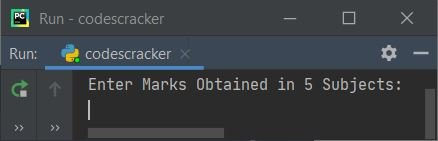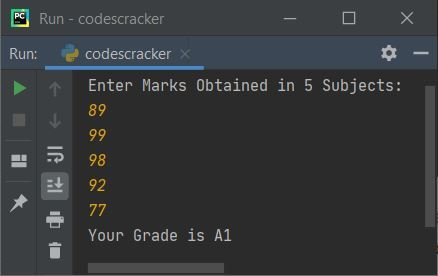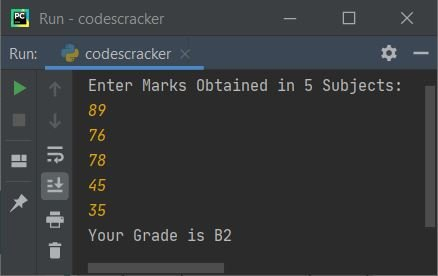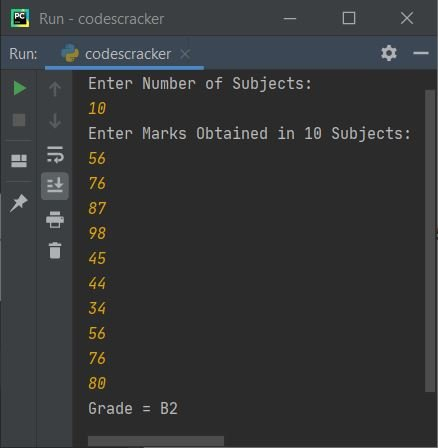# Python Program to Calculate a Student's Grade

In this article, you will learn and get code in Python to calculate the grades of students. Here is a list of programs for determining a student's grade:

• Calculate the student's grade based on the results of five subjects.
• Based on the marks obtained in N number of subjects

The grade must be calculated as per the following rules:

91-100 A1
81-90 A2
71-80 B1
61-70 B2
51-60 C1
41-50 C2
33-40 D
21-32 E1
0-20 E2

## Calculate the Grade of the Student in Python

This program figures out and prints a student's grade based on the marks they got in five subjects that the user enters at run time.

To calculate the grade of students in Python, you have to ask the user to enter marks obtained in five subjects. Now, add up all the marks and figure out the average to find the student's grade based on the average marks they got, as shown in the program below:

The following Python program asks the user to enter marks obtained in five subjects to calculate and print the student's grade:

```print("Enter Marks Obtained in 5 Subjects: ")
markOne = int(input())
markTwo = int(input())
markThree = int(input())
markFour = int(input())
markFive = int(input())

tot = markOne+markTwo+markThree+markFour+markFive
avg = tot/5

if avg>=91 and avg<=100:
elif avg>=81 and avg<91:
elif avg>=71 and avg<81:
elif avg>=61 and avg<71:
elif avg>=51 and avg<61:
elif avg>=41 and avg<51:
elif avg>=33 and avg<41:
elif avg>=21 and avg<33:
elif avg>=0 and avg<21:
else:
print("Invalid Input!")```

Here are some sample runs of the above Python program to demonstrate how to calculate the grade of a student.

When you run the above code, you will see the following sample output, which asks the user to enter marks obtained in 5 subjects:Now enter the marks obtained in 5 subjects, say 89, 99, 98, 92, and 77, and then press the ENTER key to see the grade obtained according to the marks entered, as shown in the snapshot given below:Note: If mark contains decimal values like 98.6, 86.9, etc., then replace int with float.

That is, to handle floating-point (values containing decimal) values, replace the following block of code:

```markOne = int(input())
markTwo = int(input())
markThree = int(input())
markFour = int(input())
markFive = int(input())```

with the block of code given below:

```markOne = float(input())
markTwo = float(input())
markThree = float(input())
markFour = float(input())
markFive = float(input())```

This program uses a loop to receive marks obtained in five subjects. It also uses a loop to find the total mark:

```mark = []
tot = 0
print("Enter Marks Obtained in 5 Subjects: ")
for i in range(5):
mark.insert(i, input())

for i in range(5):
tot = tot + int(mark[i])
avg = tot/5

if avg>=91 and avg<=100:
elif avg>=81 and avg<91:
elif avg>=71 and avg<81:
elif avg>=61 and avg<71:
elif avg>=51 and avg<61:
elif avg>=41 and avg<51:
elif avg>=33 and avg<41:
elif avg>=21 and avg<33:
elif avg>=0 and avg<21:
else:
print("Invalid Input!")```

Here is its sample run with user input: 89, 76, 78, 45, and 35 as marks obtained in 5 subjects:Note: You can also use append() in place of insert(). To do so, just replace the following statement:

`mark.insert(i, input())`

with the statement given below:

`mark.append(input())`

The following block of code (from the above program):

```for i in range(5):
mark.insert(i, input())```

is created to execute the following statement:

`mark.insert(i, input())`

five times from 0 to 4. That is, this statement gets executed five times with the value of i ranging from 0 to 4.

When i's value is 0 (at first execution), then the mark entered by the user gets stored in the list at mark. Now, the value of i is 1, so the mark entered by the user is stored in the list at mark, and so on up to five times.

### Obtain Marks for N Subjects and Determine Grade

This program allows the user to enter the number of subjects along with the marks obtained in all subjects. That is, this program finds and prints the grade of a student based on the marks obtained in N subjects. The value of N and marks in N subjects must be entered by the user:

```mark = []
tot = 0
print("Enter Number of Subjects: ")
subNo = int(input())
print("Enter Marks Obtained in " + str(subNo) + " Subjects: ")
for i in range(subNo):
mark.append(input())

for i in range(subNo):
tot = tot + int(mark[i])
avg = tot/subNo

if avg>=91 and avg<=100:
elif avg>=81 and avg<91:
elif avg>=71 and avg<81:
elif avg>=61 and avg<71:
elif avg>=51 and avg<61:
elif avg>=41 and avg<51:
elif avg>=33 and avg<41:
elif avg>=21 and avg<33:
elif avg>=0 and avg<21:
else:
print("Invalid Input!")```

Here is its sample run with user input, 6 as the number of subjects, and 56, 46, 76, 87, 45, and 37 as the marks obtained in the 6 subjects:Here is another sample run with user input, 10 as the total number of subjects, and 56, 76, 87, 90, 45, 44, 34, 56, 76, and 80 as the marks obtained in the 10 subjects:#### Same Program in Other Languages

Python Online Test

« Previous Program Next Program »